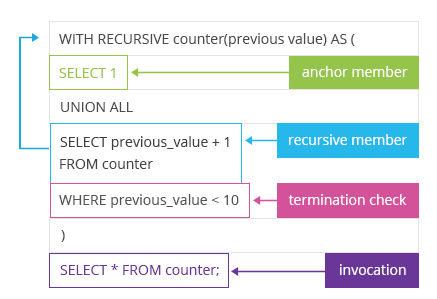Deals Of The Week - hours only!Up to 80% off on all courses and bundles.-Close
Introduction
The very basics
5. Recursion explained: syntax 1
Infinite looping
Processing trees with recursive CTEs
Processing graphs with recursive CTEs
Summary

## Instruction

Awesome! As you saw, we got rows containing the numbers from 1 to 10 without actually using any table. Now it's time to explain the magic behind that query. Take another look at the template from the previous exercise:In PostgreSQL, a recursive CTE starts with the words WITH RECURSIVE, followed by the CTE name and a list of columns in the parentheses. In this case, the CTE under the name counter has only one column, which is the previous_value (that is, the value shown in the previous step).

Every correctly constructed recursive CTE has three essential elements inside the parentheses: an anchor member, a recursive member, and a terminator. Let's discuss each of them.

An anchor member is basically something that we start with – i.e. an anchor point. As we want to show the numbers from 1 to 10, we will start with the number 1. You could think of it as the smallest matryoshka doll, which is so small that it has no other doll inside. In our case, the anchor member is expressed as:

SELECT 1

Then comes the recursive member. The recursive member tells us what to do in each recursive step. In the case of matryoshka dolls, this could be: "Create a bigger matryoshka doll, which will have the previous doll inside it".

So, for numbers, this instruction would be: "Given that you have all the rows with the numbers generated so far, add another row with a number that is bigger by 1 than the number in the row previously generated". In our case, this translates into:

SELECT previous_value + 1
FROM counter


At this point, the CTE counter refers to itself (FROM counter).

If we stopped at this point, our query would go on and on; it would never stop. In our real-life examples, each recursion comes to an end; there is the biggest matryoshka doll that holds all the smaller dolls inside itself, just as there is the biggest cone in a Romanesco broccoli. Likewise, there is a terminator in recursive CTEs: something that will eventually stop the recursion. In this case, it's:

WHERE previous_value < 10

This means: "As long as the previous value is smaller than 10, continue with the recursion. But if you reach 10, don't add any new rows". When a recursive CTE has nothing new to add in a given step, it terminates.

## Exercise

Click to continue.